# Functions for Trigonometry Chapter Exam

Exam Instructions:

Choose your answers to the questions and click 'Next' to see the next set of questions. You can skip questions if you would like and come back to them later with the yellow "Go To First Skipped Question" button. When you have completed the practice exam, a green submit button will appear. Click it to see your results. Good luck!

### Page 1

#### Question 4 4. What is the domain of the function below?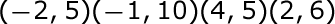#### Question 5 5. Choose the answer that best describes the function of this graph.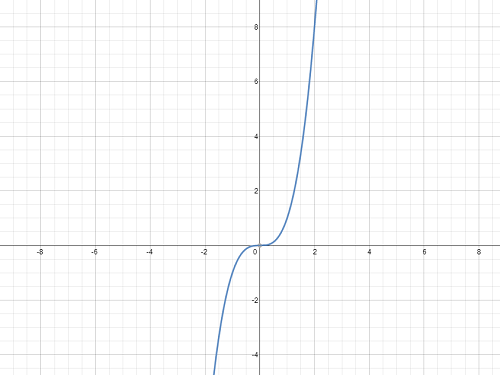### Page 2

#### Question 10 10. Choose the answer that best describes the function of this graph.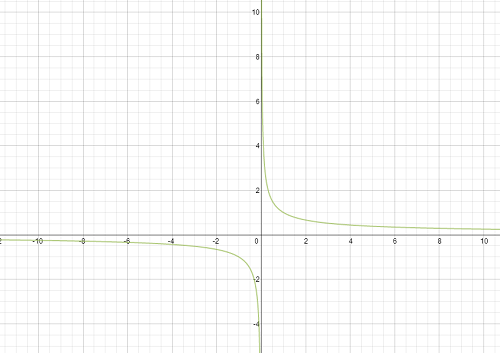### Page 3

#### Question 11 11. Which equation matches the following graph?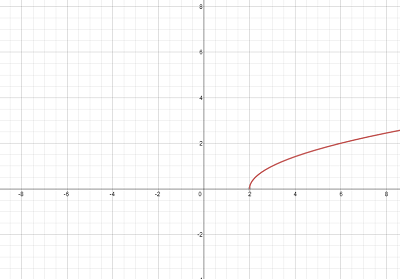#### Question 12 12. Which piecewise function is represented by this graph?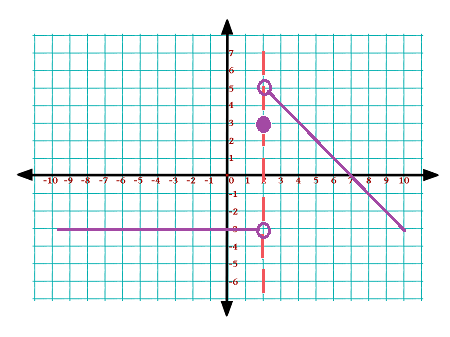#### Question 13 13. If the graph of f(x) is shown below, which choice represents the graph of f(x + 1) + 2?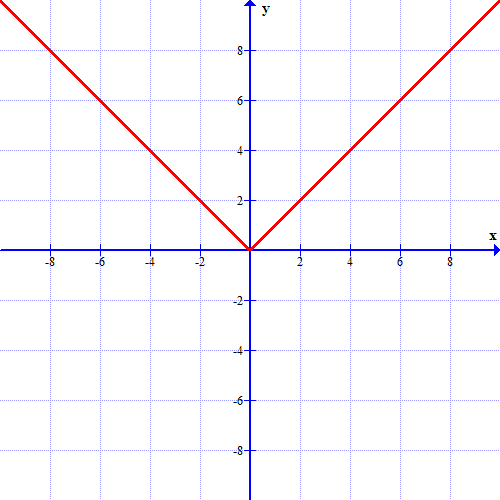### Page 4

#### Question 18 18. Choose the answer that best describes the function of this graph.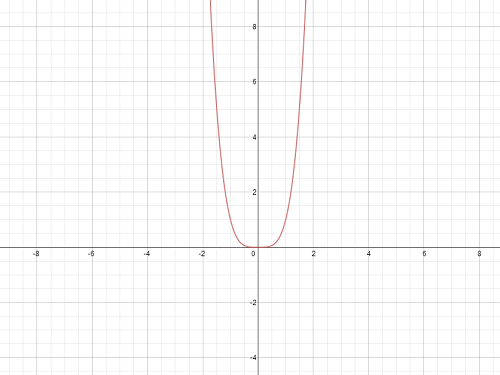#### Question 19 19. Which kind of transformation would change the purple function below into the yellow one?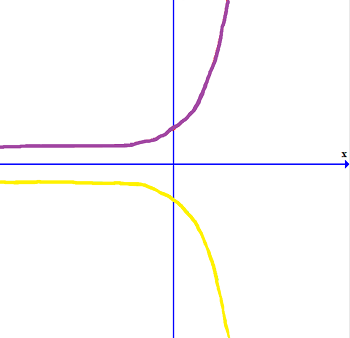### Page 5

#### Question 21 21. What is the domain of this function?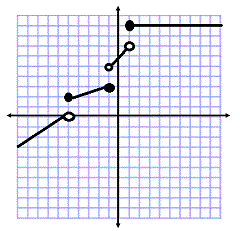#### Question 23 23. Which equation matches the graph?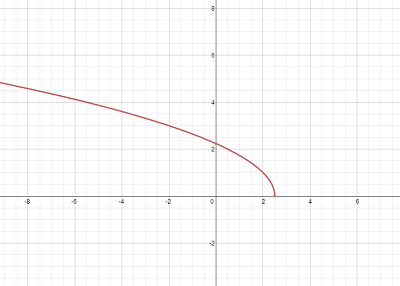### Page 6

#### Question 26 26. In the figure shown below, the graph of the yellow function is a translation of the graph of the blue function. If the expression for the blue function is y(x) = b(x), which of the following is the equation of the yellow function?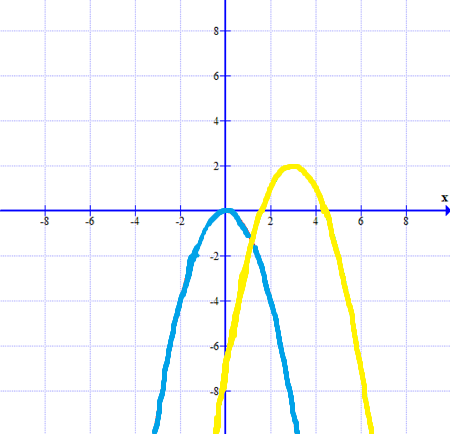#### Functions for Trigonometry Chapter Exam Instructions

Choose your answers to the questions and click 'Next' to see the next set of questions. You can skip questions if you would like and come back to them later with the yellow "Go To First Skipped Question" button. When you have completed the practice exam, a green submit button will appear. Click it to see your results. Good luck!

Support